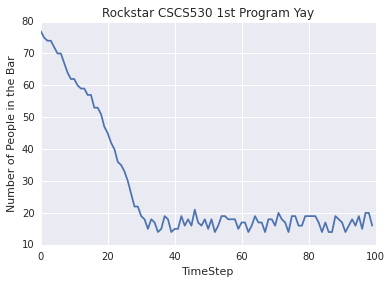## CSCS530 Winter 2015¶

#### View this repository on NBViewer¶

In :
import numpy
import matplotlib.pyplot as plt
import seaborn; seaborn.set()

# Import widget methods
from IPython.html.widgets import *

#initialNumPeople = 10 #inital number of people
#barCapacity = 80
#maxExitRatePerTimeStep = 5

#Our goal is to measure how many people are in the bar across timesteps

#create model that counts/changes number of people in the bar
#condition to stop when we reach max timestep
#Can simulate random number of people entering bar at some probability that changes over time
#based on amount over/under capacity, change probability of going to bar the next timestep
def run_simulation(initialNumPeople = 10, barCapacity=80, maxExitRatePerTimeStep = 5):
peopleList = []
minExitRatePerTimeStep = 0
maxTimeStep = 100
currentTime = 0
while currentTime < maxTimeStep:
if initialNumPeople >= barCapacity:
enteringPeeps = 0 #no one enters the bar if it is at full capacity
else:
enteringPeeps = int(numpy.random.normal(5,1))
exitingPeeps = int(numpy.random.uniform(minExitRatePerTimeStep, maxExitRatePerTimeStep))
if exitingPeeps > initialNumPeople+enteringPeeps:
exitingPeeps = 0
initialNumPeople = initialNumPeople + enteringPeeps - exitingPeeps
peopleList.append(initialNumPeople)
currentTime +=1

%matplotlib inline
f = plt.figure()
plt.plot(peopleList)
plt.xlabel('TimeStep')
plt.ylabel('Number of People in the Bar')
plt.title('Rockstar CSCS530 1st Program Yay')

# Call the ipython interact() method to allow us to explore the parameters and sampling
interact(run_simulation, initialNumPeople=(1, 200),
barCapacity = (1, 200),
maxExitRatePerTimeStep = (0, 50))
print peopleListIn [ ]: